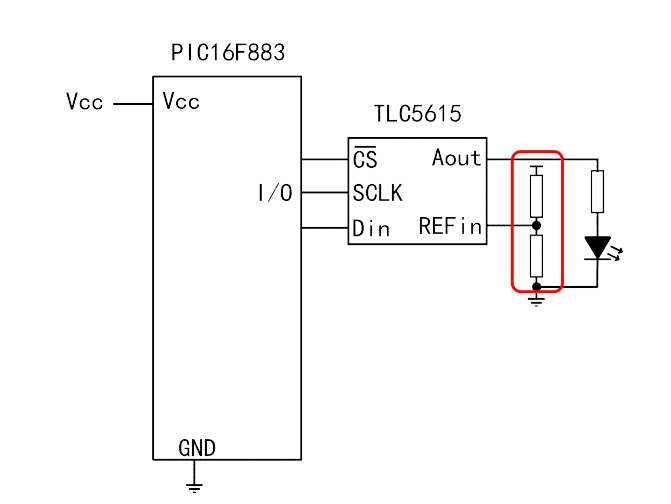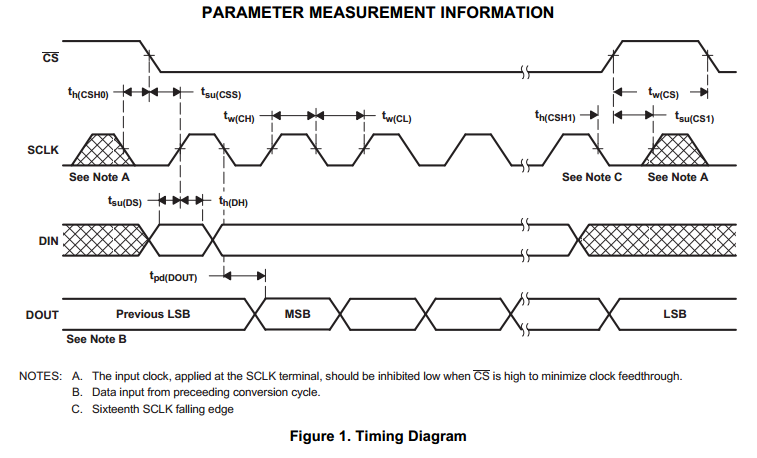#### 实验电路图：RC3连接SCLK、RC5连接Din、RC2连接CS。 输入DA的数字从0加到1024（DA为10位），再从1024减到0。中间最亮的时候做了一点儿延时。 LED灯接一个电阻到地，另一端接DA的模拟输出引脚。

#### 实验中比较重要的时序图：#### 源代码如下：

```#include<htc.h>
#define uchar unsigned char
#define uint unsigned int
#define CLK RC3
#define DATA_IN RC5
#define CS RC2
void delay(uint x)
{
uint a,b;
while(x--)
{
b = 0x00ff;
while(b--)
{
a = 0x00ff;
while(a--);
}
}
}
void main()
{
uint i,temp,k=0,kp;
TRISC=0x00;
void delay(uint x);
while(1)
{
i = 12;
CS = 0;
CLK = 0;
kp = k;
kp<<=4;
while(i--)
{
temp=kp&0x8000;
if(temp!=0)
{
DATA_IN = 1;
}
else
{
DATA_IN = 0;
}
CLK  = 1;
kp<< = 1;
CLK = 0;
}
CS  = 1;
CLK = 0;
k++;
if(k==0x0fff)
{
delay(1);
while(k--)
{
i = 12;
CS = 0;
CLK = 0;
kp = k;
kp<<=4;
while(i--)
{
temp = kp&0x8000;
if(temp!=0)
{
DATA_IN = 1;
}
else
{
DATA_IN = 0;
}
CLK  = 1;
kp<< = 1;
CLK = 0;
}
CS  = 1;
CLK = 0;
}
k = 0;
}
}
}
```